Courses

# Prestressed Concrete Civil Engineering (CE) Notes | EduRev

## Civil Engineering (CE) : Prestressed Concrete Civil Engineering (CE) Notes | EduRev

The document Prestressed Concrete Civil Engineering (CE) Notes | EduRev is a part of the Civil Engineering (CE) Course Topic wise GATE Past Year Papers for Civil Engineering.
All you need of Civil Engineering (CE) at this link: Civil Engineering (CE)

Q.1 A 6 m long simply-supported beam is prestressed as shown in the figure.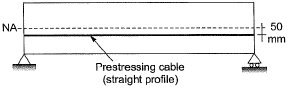The beam carries a uniformly distributed load of 6 kN/m over its entire span. If the effective flexural rigidity EI = 2 x 104 kNm2 and the effective prestressing force is 200 kN, the net increase in length of the prestressing cable (in mm, up to two decimal places) is ________ .   [2018 : 2 Marks, Set-II]
Solution:

Span of PSC beam = 6 mTotal UDL = 6 kN/m
eccentricity = e = 50 mm
(a) Slope of beam due to P-force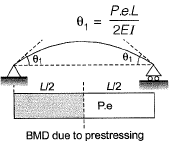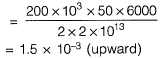(b) Slope of beam due to UDL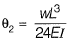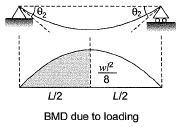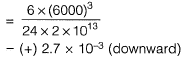(c) Net slope of beam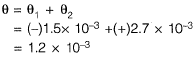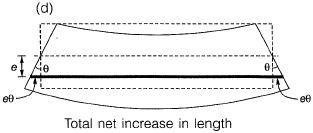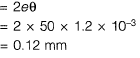Q.2 A simply supported rectangular concrete beam of span 8 m has to be prestressed with a force of 1600 kN. The tend on is of parabolic having zero eccentricity at the supports. The beam has to carry an external uniformly distributed load of intensity 30 kN/m. Neglecting the self-weight of the beam, the maximum dip (in meters, up to two decimal places) of the tendon at the mid-span to balan ce the external load should be _____ .    [2017 : 2 Marks, Set-II]
Solution: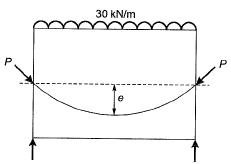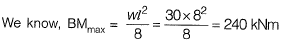Note: Tendon profile shall follow the shape of bending moment diagram.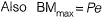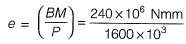e = 150 mm
e =0.15 m

Q.3 A pre-tensioned rectangular concrete beam 150 mm wide and 300 depth is prestressed with three straight tendons, each having a cross- sectional area of 50 mm2 , to an initial stress of 1200 N/mm2. The tendons are located at 100 mm from the soffit of the beam. If the modular ratio is 6, the loss of prestressing force (in kN, up to one decimal place) due to the elastic deformation of concrete only is _________.    [2017 : 2 Marks, Set-I]
Solution: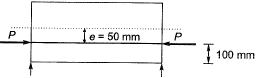Prestressing force,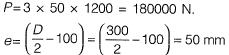Stress in concrete at the location of steel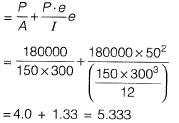Loss of stress
= m x fc = 6 x 5.3333 = 32 N/mm2
Loss of prestressing force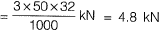Q.4 In a pre-stressed concrete beam section shown in the figure, the net loss is 10% and the final pre-stressing force applied at X is 750 kN. The initial fiber stresses (in N/mm2) at the top and bottom of the beam were:   [2015 : 2 Marks, Set-II]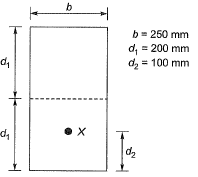(a) 4.166 and 20.833
(b) -4.166 and -20.833
(c) 4.166 and -20.833
(d) -4.166 and 20.833
Ans.
(D)
Solution:
Given, net loss = 10%
Final prestressing force after loss = 750 kN
Hence, initial prestressing force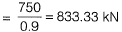Initial stresses at top and bottom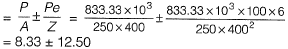Stress at top = 8.33 - 12.50 = - 4.17 (tensile)
Stress at bottom = 8.33 + 12.50 = 20.83 (Comp.)

Q.5 A rectangular concrete beam 250 mm wide and 600 mm deep is prestressed by means of 16 wire of high tensile steel wires, each of 7 mm dia. located at 200 mm from the bottom face of the beam at a given section. If the effective prestress in the wires is 700 MPa. What is the maximum sagging BM (in kN-m) due to live load which this section of the beam can withstand without causing tensile stresses at the bottom face of the beam. Neglect the self weight of the beam.   [2013 : 2 Marks]
Solution:

Since the tensile stress at bottom face of the beam is zero.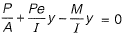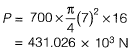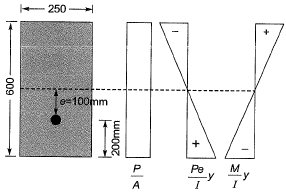Since the prestressing force is located at 200 mm from the bottom face of the beam.
∴ Eccentricity = 300 - 200 = 100 mm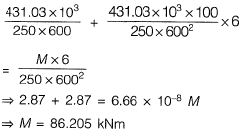Q.6 Which one of the following is categorised as a long-term loss of prestresss in a pre-stressed concrete member?    [2012 : 1 Mark]
(a) Loss due to elastic shortening
(b) Loss due to friction
(c) Loss due to relaxation of strands
(d) Loss due to anchorage slip
Ans. (C)
Solution: Loss due to relaxation, shrinkage of concrete and due to creep are long term losses.

Q.7 A concrete beam pre-stressed with a parabolic tendon is shown in the sketch. The eccentricity of the tendon is measured from the centroid of the cross-section. The applied pre-stressing force at service is 1620 kN. The uniformly distributed load of 45 kN/m includes the self-weight.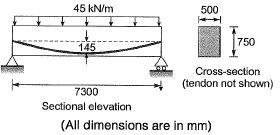The stress (in N/mm2) in the bottom fibre at midspan is    [2012 : 2 Marks]
(a) tensile 2.90
(b) compressive 2.90
(c) tensile 4.32
(d) compressive 4.32
Ans.
(B)
Solution: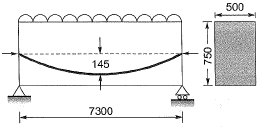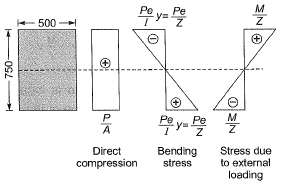Total stress at Bottom =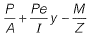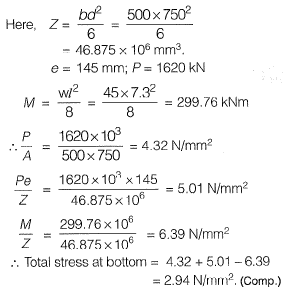Offer running on EduRev: Apply code STAYHOME200 to get INR 200 off on our premium plan EduRev Infinity!

69 docs

,

,

,

,

,

,

,

,

,

,

,

,

,

,

,

,

,

,

,

,

,

;